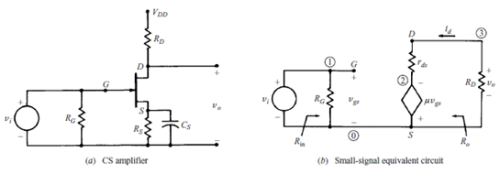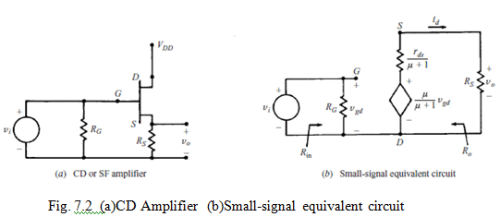FET Amplifier

# FET Amplifier Notes | Study Analog Electronics - Electrical Engineering (EE)

## Document Description: FET Amplifier for Electrical Engineering (EE) 2022 is part of FET Amplifier for Analog Electronics preparation. The notes and questions for FET Amplifier have been prepared according to the Electrical Engineering (EE) exam syllabus. Information about FET Amplifier covers topics like and FET Amplifier Example, for Electrical Engineering (EE) 2022 Exam. Find important definitions, questions, notes, meanings, examples, exercises and tests below for FET Amplifier.

Introduction of FET Amplifier in English is available as part of our Analog Electronics for Electrical Engineering (EE) & FET Amplifier in Hindi for Analog Electronics course. Download more important topics related with FET Amplifier, notes, lectures and mock test series for Electrical Engineering (EE) Exam by signing up for free. Electrical Engineering (EE): FET Amplifier Notes | Study Analog Electronics - Electrical Engineering (EE)
 1 Crore+ students have signed up on EduRev. Have you?

INTRODUCTION

Field Effect Transistor (FET) amplifiers provide an excellent voltage gain and high input impedance. Because of high input impedance and other characteristics of JFETs they are preferred over BJTs for certain types of applications.

There are 3 basic FET circuit configurations:

i)Common Source

ii)Common Drain

iii)Common Gain

Similar to BJT CE, CC and CB circuits, only difference is that in BJT large output collector current is controlled by small input base current whereas FET controls output current by means of small input voltage. In both the cases output current is a controlled variable.

FET amplifier circuits use voltage controlled nature of the JFET. In Pinch-off region, ID depends only on VGS.

Common Source (CS) AmplifierFig. 7.1 (a) CS Amplifier (b) Small-signal equivalent circuit

A simple Common Source amplifier is shown in Fig. 7.1(a) and associated small signal equivalent circuit using voltage-source model of FET is shown in Fig. 7.1(b)

Voltage Gain

Source resistance (RS) is used to set the Q-Point but is bypassed by CS for mid-frequency operation. From the small signal equivalent circuit ,the output voltage

VO = -RDµVgs(R+ rd)

Where Vgs = Vi , the input voltage,

Hence, the voltage gain,

AV = V/ Vi = -RDµ(R+ rd)

Input Impedance

From Fig. 7.1(b) Input Impedance is

Zi =  RG

For voltage divider bias as in CE Amplifiers of BJT

RG =  R1 ║ R

Output Impedance

Output impedance is the impedance measured at the output terminals with the input voltage V­I = 0

From the Fig. 7.1(b) when the input voltage V= 0, Vgs = 0 and hence

µ Vgs = 0

The equivalent circuit for calculating output impedance is given in Fig. 7.2.

Output impedance  Z= rd ║ RD

Normally rd will be far greater than RD . Hence Z≈ RD

Common Drain Amplifier

A simple common drain amplifier is shown in Fig. 7.2(a) and associated small signal equivalent circuit using the voltage source model of FET is shown in Fig. 7.2(b). Since voltage Vgd is more easily determined than Vgs, the voltage source in the output circuit is expressed in terms of Vgs and Thevenin’s theorem.Voltage Gain

The output voltage,

VO = R­SµVgd / (µ + 1) R­S + rd

Where        Vgd = Vi  the input voltage.

Hence, the voltage gain,

Av = VO / Vi = R­Sµ / (µ + 1) R­S + rd

Input Impedance

From Fig. 7.2(b), Input Impedance Z­= RG

Output Impedence

From Fig. 7.2(b), Output impedance measured at the output terminals with input voltage Vi = 0 can be calculated from the following equivalent circuit.

As Vi = 0: Vgd = 0:  µvgd  / (µ + 1) = 0

Output Impedance

Z= rd / (µ + 1) ║RS

When  µ » 1

ZO = ( rd / µ) ║RS = (1/gm) ║RS

The document FET Amplifier Notes | Study Analog Electronics - Electrical Engineering (EE) is a part of the Electrical Engineering (EE) Course Analog Electronics.
All you need of Electrical Engineering (EE) at this link: Electrical Engineering (EE)

## Analog Electronics

11 videos|57 docs|97 tests
 Use Code STAYHOME200 and get INR 200 additional OFF

## Analog Electronics

11 videos|57 docs|97 tests

Track your progress, build streaks, highlight & save important lessons and more!

,

,

,

,

,

,

,

,

,

,

,

,

,

,

,

,

,

,

,

,

,

;